Practice Test: Civil Engineering (CE)- 4

# Practice Test: Civil Engineering (CE)- 4 - Civil Engineering (CE)

Test Description

## 65 Questions MCQ Test GATE Civil Engineering (CE) 2023 Mock Test Series - Practice Test: Civil Engineering (CE)- 4

Practice Test: Civil Engineering (CE)- 4 for Civil Engineering (CE) 2023 is part of GATE Civil Engineering (CE) 2023 Mock Test Series preparation. The Practice Test: Civil Engineering (CE)- 4 questions and answers have been prepared according to the Civil Engineering (CE) exam syllabus.The Practice Test: Civil Engineering (CE)- 4 MCQs are made for Civil Engineering (CE) 2023 Exam. Find important definitions, questions, notes, meanings, examples, exercises, MCQs and online tests for Practice Test: Civil Engineering (CE)- 4 below.
Solutions of Practice Test: Civil Engineering (CE)- 4 questions in English are available as part of our GATE Civil Engineering (CE) 2023 Mock Test Series for Civil Engineering (CE) & Practice Test: Civil Engineering (CE)- 4 solutions in Hindi for GATE Civil Engineering (CE) 2023 Mock Test Series course. Download more important topics, notes, lectures and mock test series for Civil Engineering (CE) Exam by signing up for free. Attempt Practice Test: Civil Engineering (CE)- 4 | 65 questions in 180 minutes | Mock test for Civil Engineering (CE) preparation | Free important questions MCQ to study GATE Civil Engineering (CE) 2023 Mock Test Series for Civil Engineering (CE) Exam | Download free PDF with solutions
 1 Crore+ students have signed up on EduRev. Have you?
Practice Test: Civil Engineering (CE)- 4 - Question 1

### Choose the word or phrase which is nearest in meaning to the key word:             Pilfer

Practice Test: Civil Engineering (CE)- 4 - Question 2

### The following question comprises two words that have a certain relationship between them followed by four pairs of words. Select the pair that has same relationship as the original pair of words             Fox : Cunning

Practice Test: Civil Engineering (CE)- 4 - Question 3

### Replace the phrase printed in bold to make it grammatically correct ?   A twenty-first century economy "cannot be held" hostage by power cuts nor travel on nineteenth century roads.

Practice Test: Civil Engineering (CE)- 4 - Question 4

Improve the sentence with suitable options by replacing the underlined word.

He "lay" on the grass enjoying the sunshine.

Practice Test: Civil Engineering (CE)- 4 - Question 5

Fill in the blanks :

At times, we are all ______ to be mistaken.

Practice Test: Civil Engineering (CE)- 4 - Question 6

There are two solutions of wine and water, the concentration of wine being 0.4 and 0.6 respectively. If five liters of the first solution be mixed with fifteen liters of the second, find the concentration of wine in the resultant solution.

Detailed Solution for Practice Test: Civil Engineering (CE)- 4 - Question 6

Resultant concentration of wine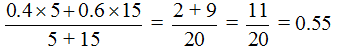Practice Test: Civil Engineering (CE)- 4 - Question 7

Rajiv bought some apples at the rate of 25 for Rs. 400 and an equal number at the rate of 30 for Rs. 270. He then sold all at the rate of 10 for Rs. 180. Find his profit/loss percentage

Detailed Solution for Practice Test: Civil Engineering (CE)- 4 - Question 7

Let Rajiv bought x apples of each kind.

Total cost price of Rajiv =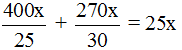Total selling price of Rajiv =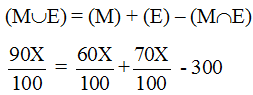SP > CP , Hence he makes profit;

Profit percentage =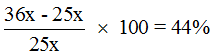Practice Test: Civil Engineering (CE)- 4 - Question 8

Out of 10 persons working on a project, 4 are graduates. If 3 are selected, what is the probability that there is alteast one graduate among them ?

Detailed Solution for Practice Test: Civil Engineering (CE)- 4 - Question 8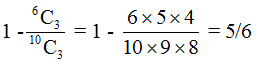Practice Test: Civil Engineering (CE)- 4 - Question 9

2 men and 3 boys can do a piece of work in 10 days, while 3 men and 2 boys can do the same work in 8 days. In how many days can 2 men and 1 boy do the work ?

Detailed Solution for Practice Test: Civil Engineering (CE)- 4 - Question 9

Let work done by 1 man in 1 day = x units

Let work done by 1 boy in 1 day = y units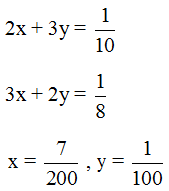2 men and 1 boy can finish work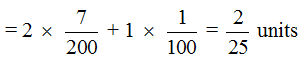No. of days taken by 2 men and 1 boy = = 12.5 days

Practice Test: Civil Engineering (CE)- 4 - Question 10

In an exam, 60% of the candidates passed in Maths and 70% candidates passed in English and 10% of the candidates failed in both the subjects, 300 candidates passed in both the subjects. Find the total number of candidates appeared in the exam, if they took test in only two subjects that is Math and English.

Detailed Solution for Practice Test: Civil Engineering (CE)- 4 - Question 10

Let total number of students appear be X.X = 750

Practice Test: Civil Engineering (CE)- 4 - Question 11

A major requirement of GIS application is :

Practice Test: Civil Engineering (CE)- 4 - Question 12

Practice Test: Civil Engineering (CE)- 4 - Question 13

In a particular bank 60% of the customers applied for a loan are rejected. If 4 new loan applications are received, find the probability that exactly 3 of them are accepted.

Detailed Solution for Practice Test: Civil Engineering (CE)- 4 - Question 13

n = 4, p = 0.4, q = 0.6
Applying Binomial theorem:

p(X = 3) =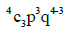p(X = 3) =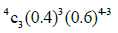= 0.1536

Practice Test: Civil Engineering (CE)- 4 - Question 14

The following zones formed in polluted river:
1.Zone of clear water
2.Zone of active decomposition
3.Zone of recovery
The correct sequence in which these zones occur progressively downstream in a polluted river is:

Practice Test: Civil Engineering (CE)- 4 - Question 15

The static indeterminacy of given structure is :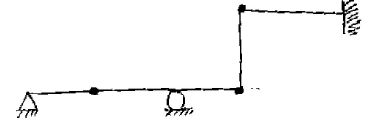Detailed Solution for Practice Test: Civil Engineering (CE)- 4 - Question 15

Ds = 0

Practice Test: Civil Engineering (CE)- 4 - Question 16

The structure is carrying a horizontal load P at E. Legs AB and CD are vertical. The stress resultants in AB segment are :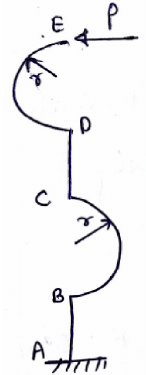Practice Test: Civil Engineering (CE)- 4 - Question 17

For f(x) = ex(sin x − cos x) such that x ε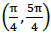, find the value of c by applying mean value theorem.

Detailed Solution for Practice Test: Civil Engineering (CE)- 4 - Question 17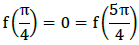f ´(x) = ex(cos x sin x) ex(sin x − cos x)
2ex sin x = 0
2ec sin c = 0
sin c = sin π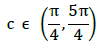c = π

Practice Test: Civil Engineering (CE)- 4 - Question 18

If all the dimensions of a vertically subtended circular bar are doubled, then the maximum stress produced in it due to its own weight will :

Detailed Solution for Practice Test: Civil Engineering (CE)- 4 - Question 18

Maxm. Stress at point where full weight acts.
Stress =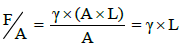Finally, stress = γ x 2L

Stress will be doubled.

Practice Test: Civil Engineering (CE)- 4 - Question 19

In beam ABCD, there is a roller support at B and C as shown in the figure. If 200 kN load is applied at the mid span of BC. The shear force at the support A will be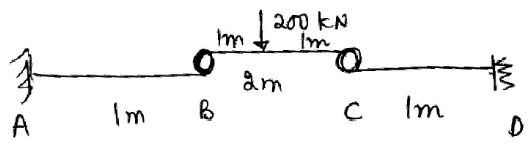Detailed Solution for Practice Test: Civil Engineering (CE)- 4 - Question 19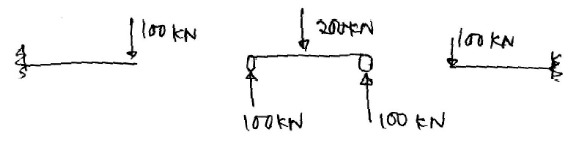SF at a = 100 kN

Practice Test: Civil Engineering (CE)- 4 - Question 20

For a given storm the average depth of rainfall over an area:

Practice Test: Civil Engineering (CE)- 4 - Question 21

For a steel cube shown in the figure, the net volume change after loading is found to be zero. The magnitude of σ will be ? (Given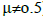)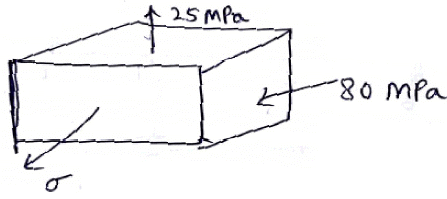Detailed Solution for Practice Test: Civil Engineering (CE)- 4 - Question 21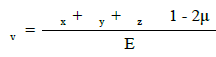If v = 0

σ x  σ y σz = 0
25 – 80  σ = 0
σ= 55 MPa

Practice Test: Civil Engineering (CE)- 4 - Question 22

Flow separation is caused by :

Detailed Solution for Practice Test: Civil Engineering (CE)- 4 - Question 22

Practice Test: Civil Engineering (CE)- 4 - Question 23

Determine the rate of flow in the figure given below :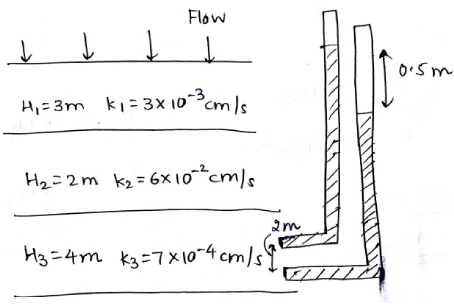Detailed Solution for Practice Test: Civil Engineering (CE)- 4 - Question 23

q = k i A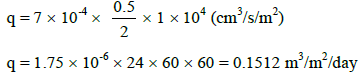Practice Test: Civil Engineering (CE)- 4 - Question 24

A soil sampler has inner and outer radii of 28 mm and 34 mm respectively. The area ratio of the sampler is

Detailed Solution for Practice Test: Civil Engineering (CE)- 4 - Question 24

Area ratio (Ar)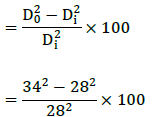= 48%

Practice Test: Civil Engineering (CE)- 4 - Question 25

A D-hour unit hydrograph differs from a 2-D hour unit hydrograph for any catchment is that:

Practice Test: Civil Engineering (CE)- 4 - Question 26

The flow in a channel is at critical depth. If at a section M a small hump of height Δz is built on a bed of the channel, the flow will be:

Practice Test: Civil Engineering (CE)- 4 - Question 27

The determination of ultimate bearing capacity of an eccentrically loaded square footing depends upon the concept of useful:

Practice Test: Civil Engineering (CE)- 4 - Question 28

The sensitivity of a level tube decreases if:

Detailed Solution for Practice Test: Civil Engineering (CE)- 4 - Question 28

Sensitivity of a level tube decreases if diameter of the tube is decreased.

Practice Test: Civil Engineering (CE)- 4 - Question 29

The nominal bearing strength of the bolt is given by 2.5 Kb dt fu, where Kb depends upon:
1) Edge distance
2) Bolt diameter
3) Diameter of bolt hole
4) Ultimate tensile stress of bolt
5) Ultimate tensile stress of plate Of the above

Detailed Solution for Practice Test: Civil Engineering (CE)- 4 - Question 29

Kb does not depend on bolt diameter.

Practice Test: Civil Engineering (CE)- 4 - Question 30

The flow characteristics before and after a hydraulic jump on horizontal frictionless channel are such that:

Practice Test: Civil Engineering (CE)- 4 - Question 31

Consider the following statements regarding the plane truss :

(1) If 2J > m γ , the truss is always unstable.
(2) If 2J = m γ , the truss is statically determined and stable.
(3) If 2J < m γ , the truss is statically indeterminate and stable.
Of these statements :

Detailed Solution for Practice Test: Civil Engineering (CE)- 4 - Question 31

2J m γ does not ensure stability. For stability in this case the structure need to be inspected.

Practice Test: Civil Engineering (CE)- 4 - Question 32

For an activity i-j the early event times at i and j and late event times at i and j respectively are 5, 24, 9 and 29. The activity duration is 6. The independent float of activity i-j is :

Practice Test: Civil Engineering (CE)- 4 - Question 33

A construction concrete mixer of capacity 0.6m3 is used and the miner discharges the entire batch of concrete into a single hopper. The time per cycle may be taken for charging, mixing and discharging mixes as 1.6 minutes and the lost time may be taken as 0.2 minutes. The number of batches per hour is equal to

Detailed Solution for Practice Test: Civil Engineering (CE)- 4 - Question 33

Number of batches per hour =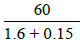Practice Test: Civil Engineering (CE)- 4 - Question 34

Which one of the following correctly expresses the split tensile strength of a circular cylinder of length L and diameter D subjected to a maximum load of P ?

Practice Test: Civil Engineering (CE)- 4 - Question 35

The base width of solid gravity dam is 25m. The material of the dam has a specific gravity of 2.56 and the dam is designed as an elementary profile ignoring uplift. Find the allowable height of the dam (in m).

Detailed Solution for Practice Test: Civil Engineering (CE)- 4 - Question 35

For elementary profile,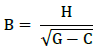For no uplift, C = 0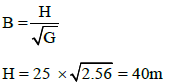Practice Test: Civil Engineering (CE)- 4 - Question 36

Consider a system of two identical particles. One of the particle is at rest and the other has an acceleration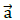.The centre of mass has an acceleration.

Detailed Solution for Practice Test: Civil Engineering (CE)- 4 - Question 36

Let acceleration of centre of mass be acm
(2m) acm = m×a ma
acm = a/2

Practice Test: Civil Engineering (CE)- 4 - Question 37

Two small kids weighing 10kg and 15kg respectively are trying to balance a see-saw of total length 5m with the fulcrum at the centre. If one of the kids weighing 10kg is sitting at an end, at what distance from the other end should the other kid it?

Detailed Solution for Practice Test: Civil Engineering (CE)- 4 - Question 37

Supposing the other kid to be seated at a distance x from the free end
10 × 9.81 × 2.5 = 15 × (2.5 - x) × 9.81
x = 0.83m

Practice Test: Civil Engineering (CE)- 4 - Question 38

A sample of ground water at a pH of 8 contains 122 mg/L of bicarbonates. What is the alkalinity of this water (in terms of CaCO3)?

Detailed Solution for Practice Test: Civil Engineering (CE)- 4 - Question 38

Concentration of [OH¯] is very less. Hence Alkalinity expressed as CaCO3 =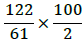= 100 mg/L

Practice Test: Civil Engineering (CE)- 4 - Question 39

Influence line diagram for axial force in member HI will be (assume compressive force as positive):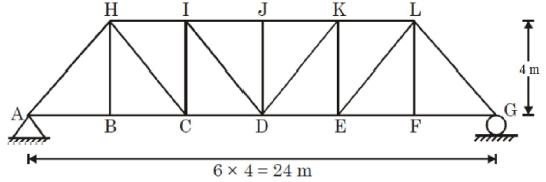Detailed Solution for Practice Test: Civil Engineering (CE)- 4 - Question 39

ILD for member HI will be: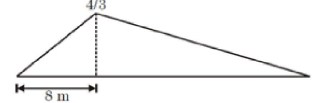Practice Test: Civil Engineering (CE)- 4 - Question 40

For the beam shown in the figure below what is the influence line diagram for moment at A?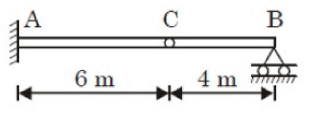Practice Test: Civil Engineering (CE)- 4 - Question 41

The staff reading taken from an instrument when bubble was at center on a staff kept at 350 m distance was 2.62m. The bubble was then moved by 8 divisions and staff
reading changed to 2.95m. If length of one division of bubble tube is 2mm. Calculate the sensitivity of a bubble tube.

Detailed Solution for Practice Test: Civil Engineering (CE)- 4 - Question 41

Length of one division = 2mm
n = divisions, D = 350m
Staff intercept, S = S2 – S1 = 2.95 - 2.62 = 0.33m

Sensitivity of the bubble tube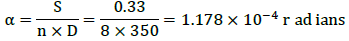α = 24.3 seconds

Practice Test: Civil Engineering (CE)- 4 - Question 42

Two people weighing W each are sitting on a plank of length L floating on water at L/3 from either end. Neglecting the weight of the plank, the bending moment and shear at the center of plank is:

Detailed Solution for Practice Test: Civil Engineering (CE)- 4 - Question 42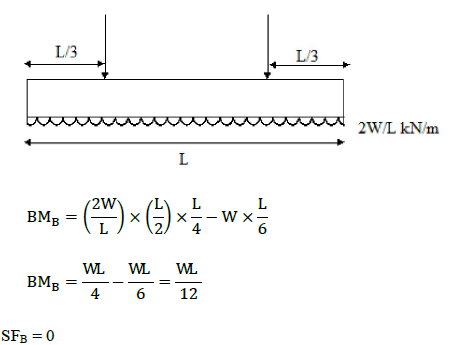Practice Test: Civil Engineering (CE)- 4 - Question 43

The reaction R in the given figure is: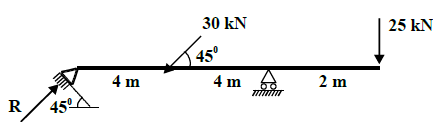Detailed Solution for Practice Test: Civil Engineering (CE)- 4 - Question 43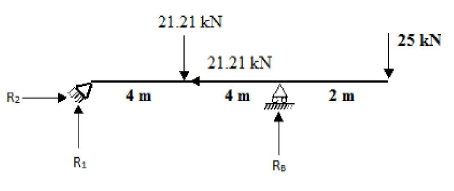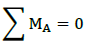−25 × 10 RB × 8 − 21.21 × 4 = 0
RB = 41.855 kN
R1 = 21.21 25 − 41.855 = 4.355 kN
R2 = 21.21 kN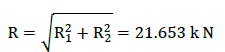Practice Test: Civil Engineering (CE)- 4 - Question 44

The average annual rainfall in cm at four existing rain gauge stations in basin is 105, 79, 70 and 66. If the average depth of rainfall over the basin is to be estimated within 10% error, determine the number of gauges needed.

Detailed Solution for Practice Test: Civil Engineering (CE)- 4 - Question 44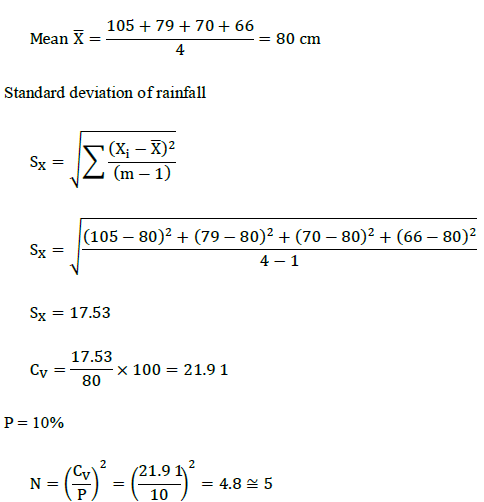Practice Test: Civil Engineering (CE)- 4 - Question 45

For a catchment area of 72 km2 the equilibrium discharge of an S curve obtained by 2 hour unit hydrograph summation is:

Detailed Solution for Practice Test: Civil Engineering (CE)- 4 - Question 45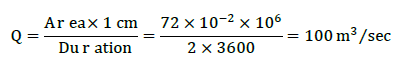Practice Test: Civil Engineering (CE)- 4 - Question 46

The function f(x) is given by: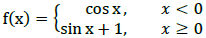Detailed Solution for Practice Test: Civil Engineering (CE)- 4 - Question 46f(0-) = f(0 ), Hence f(x) is continuous at x = 0
f `(0-) = − sin(0) = 0
f `(0 ) = cos(0) = 0
Hence, f(x) is continuous but not differentiable at x = 0

Practice Test: Civil Engineering (CE)- 4 - Question 47

The value of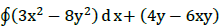dy, where c is the region bounded by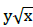and y = x2.

Detailed Solution for Practice Test: Civil Engineering (CE)- 4 - Question 47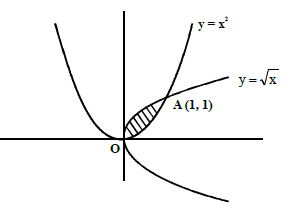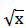= = x2 ⇒ x4  = x

x3(x3 − 1) = 0
x = 0, 1
y = 0, 1

Hence y =and y = x2 coincide at (0, 0) and (1, 1)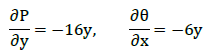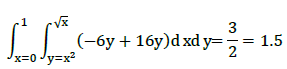Practice Test: Civil Engineering (CE)- 4 - Question 48

Find the value of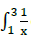dx  by Simpson’s 1/3rd  rule. Take (n = 2).

Detailed Solution for Practice Test: Civil Engineering (CE)- 4 - Question 48Practice Test: Civil Engineering (CE)- 4 - Question 49

A sample of sand has a volume of 1000mL in its natural state. Its volume when compacted is 840 mL. When gently poured in a measuring cylinder its maximum volume is 1370 mL, the relative density of sand is ?

Detailed Solution for Practice Test: Civil Engineering (CE)- 4 - Question 49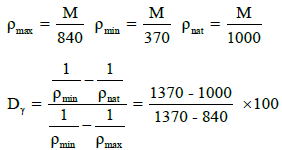= 69.81%

Practice Test: Civil Engineering (CE)- 4 - Question 50

A slope is to be constructed at an angle of 300 with the horizontal. The required factor of safety with respect to both cohesion and angle of internal friction is 1.5 and the soil has following properties.C = 10 kN/m2, φ = 22.50 and ρ = 20 kN/m3 ,Taylor’s stability numbers for mobilized friction angles of 22.50 and 150 are 0.016 and 0.046 respectively. Safe height of slope is…………..m

Detailed Solution for Practice Test: Civil Engineering (CE)- 4 - Question 50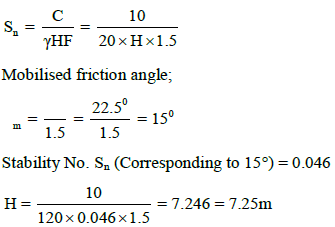Practice Test: Civil Engineering (CE)- 4 - Question 51

Three point loads of magnitude 20 kN, 40 kN and 60 kN crosses the simply supported beam AB of span 18m from left to right as shown below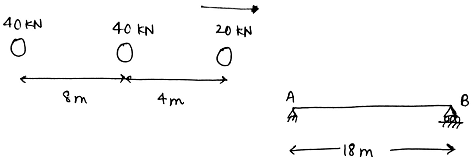If absolute maximum bending moment occurs at ‘x’ m from left end A and magnitude of absolute bending moment is y kNm, then the value of y/x is :

Detailed Solution for Practice Test: Civil Engineering (CE)- 4 - Question 51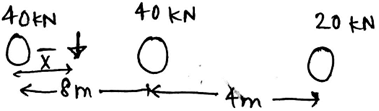Let resultant of this load system acts at a distance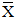from 40 kN load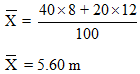Absolute maximum bending moment occurs at pt Q as shown :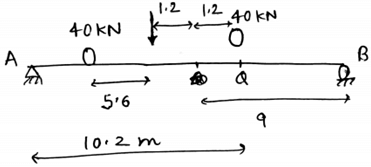Hence Mc = y = 258 kNm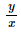= 25.29

Practice Test: Civil Engineering (CE)- 4 - Question 52

If a circular arch AB of radius R is fixed at end A and free at end B and concentrated moment is applied at end B. If flexural rigidity of arch is EI. The vertical deflection of point B will be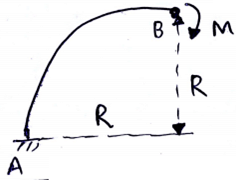Detailed Solution for Practice Test: Civil Engineering (CE)- 4 - Question 52

Applying strain energy method: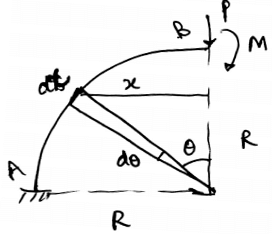BM at given pt = -M – Px

x = R Sin θ

Mx = -(M PR Sin θ)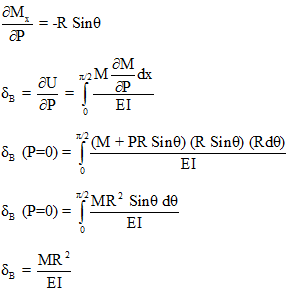Practice Test: Civil Engineering (CE)- 4 - Question 53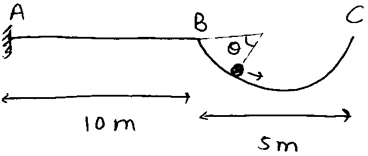Portion BC is semi-circulate. If after several oscillation ball of mass 10 Kg comes to rest. The bending moment at A when ball is at rest will be ? Take g = 9.81 m/s2.

Detailed Solution for Practice Test: Civil Engineering (CE)- 4 - Question 53

At rest, ball is at lower most potion of  semi-circle.

BM at A                    =    10g ´ (10 2.5)

=    10 ´ 9.81 ´ 12.5

=    1226.25 N - m

Practice Test: Civil Engineering (CE)- 4 - Question 54

A flat steel bar with constant EI and length L is bent by couples at the ends so that the midpoint deflection is δ (δ<<L). Then the value of applied couple M is ?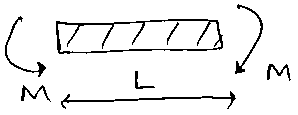Detailed Solution for Practice Test: Civil Engineering (CE)- 4 - Question 54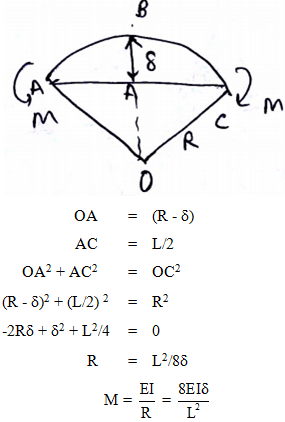Practice Test: Civil Engineering (CE)- 4 - Question 55

A prismatic bar AB of solid circular cross-section (diameter-d) is loaded by a distributed torque. The intensity of the torque, that is, the torque per unit distance, is denoted by t(x) and varies linearly from maximum value tA at end A to zero at end B. Also the length of bar is L and shear modulus of elasticity of material is G.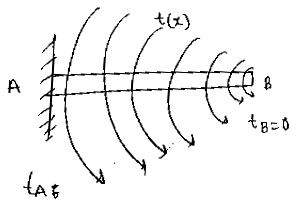What is the maximum shear stress τmax in the bar ?

Detailed Solution for Practice Test: Civil Engineering (CE)- 4 - Question 55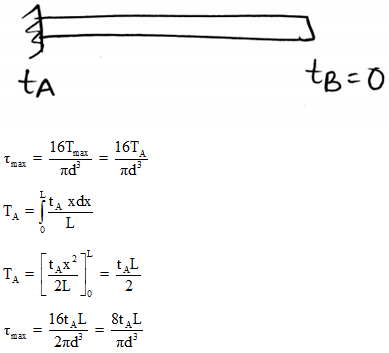Practice Test: Civil Engineering (CE)- 4 - Question 56

A beam carrying a uniformly distributed load rests on two supports ‘b’ apart with equal overhangs ‘a’ at each end. The ratio b/a for zero bending moment at mid-span is.

Detailed Solution for Practice Test: Civil Engineering (CE)- 4 - Question 56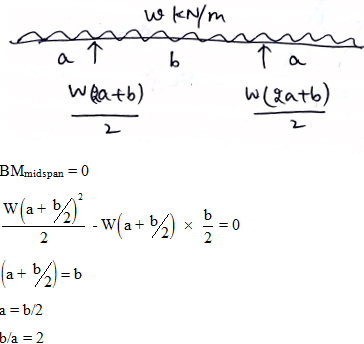Practice Test: Civil Engineering (CE)- 4 - Question 57

The bending moment at the mid-span location x-x in the beam with overhangs shown below is equal to: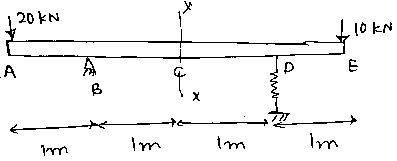Detailed Solution for Practice Test: Civil Engineering (CE)- 4 - Question 57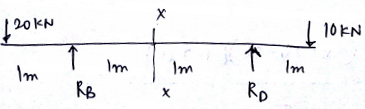RB RD = 30 kN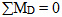RB x 2 – 20 x 3 10 x 1 = 0

R= 25 kN

R= 5 kN

BM at x – x = 25 x 1 – 20 x 2 = -15 kNm

Practice Test: Civil Engineering (CE)- 4 - Question 58

A closed tank containing water is partly filled with water and the air space above it is under pressure. A 5cm hose connected to the tank discharges water to atmosphere on to the roof of a building 3m above the level of water in the tank. If friction losses are 1.5m of water, what air pressure must be maintained in the tank to deliver 15L/s to the roof ?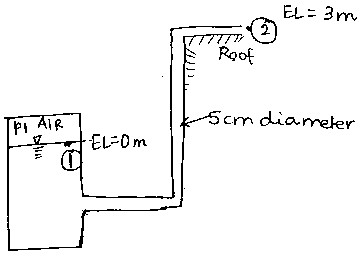Detailed Solution for Practice Test: Civil Engineering (CE)- 4 - Question 58

Q = 0.015 m3/sec =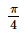x (0.05)2 x V2

V2 = 7.639 m/s

By applying Bernoulli equation to point 1 and 2;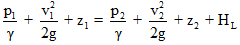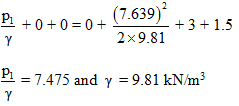p1 = 73.33 kPa

Practice Test: Civil Engineering (CE)- 4 - Question 59

In a pipe flow, the head lost due to friction is 6m. If the power transmitted through the pipe has to be maximum then the total head at the inlet will have to be maintained at :

Detailed Solution for Practice Test: Civil Engineering (CE)- 4 - Question 59

Head lost due to friction is 6m. Power transmitted is maximum when the friction head is 1/3rd of the supply head.

Practice Test: Civil Engineering (CE)- 4 - Question 60

A fluid jet is discharging from a 100mm nozzle and the vena contracta formed has a diameter 90mm. If the coefficient of velocity is 0.95, then the coefficient of discharge for the nozzle is :

Detailed Solution for Practice Test: Civil Engineering (CE)- 4 - Question 60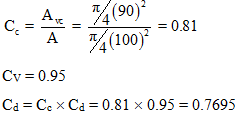Practice Test: Civil Engineering (CE)- 4 - Question 61

Find the strain energy stored by the loaded truss shown in the figure. AE = constant.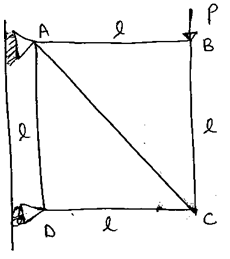Detailed Solution for Practice Test: Civil Engineering (CE)- 4 - Question 61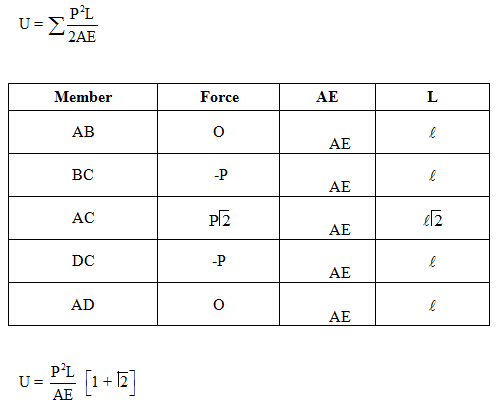Practice Test: Civil Engineering (CE)- 4 - Question 62

A cantilever beam BD rests on a simply supported beam as shown in the figure. Both the beams are of same material and are 3m wide and 8m deep if they jointly carry a load P = 1400 kN, the moment developed at D is ?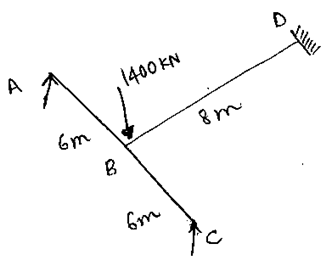Detailed Solution for Practice Test: Civil Engineering (CE)- 4 - Question 62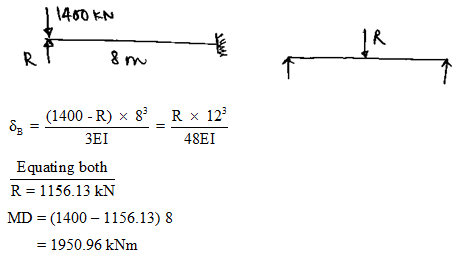Practice Test: Civil Engineering (CE)- 4 - Question 63

The average flow of traffic on cross roads A and B, both 2 lane, during design period are 850 PCU per hour and 500 PCU per hour respectively. The all red time required for pedestrian crossing is 12 seconds. The saturation headway is 2.3 seconds. Maxm . Green time available on the 2 phase is …………. Seconds.

Detailed Solution for Practice Test: Civil Engineering (CE)- 4 - Question 63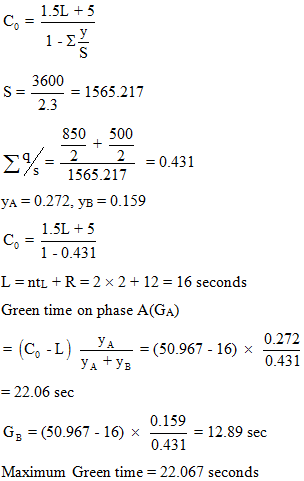Practice Test: Civil Engineering (CE)- 4 - Question 64

In Marshall method of mix design, the course aggregate, fine aggregated, filler and bitumen having respective specific gravities of 2.6, 2.7, 2.65 and 1.02 are mixed in the ratio of 0.55 : 0.346 : 0.48 : 0.056 respectively.

Specific gravity of the mix would be………..

Detailed Solution for Practice Test: Civil Engineering (CE)- 4 - Question 64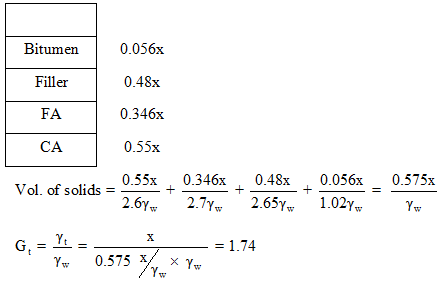Practice Test: Civil Engineering (CE)- 4 - Question 65

Beam ACB hangs from 2 springs as shown in the figure. The springs have stiffness K1 and K2 and the beam has flexural rigidity EI. Deflection of point C is :

M0       =       10 kNm

K1     =    250 kN/m

K2     =    160 kN/m

L       =    1.8 m

EI     =    216 kNm2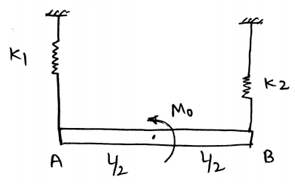Detailed Solution for Practice Test: Civil Engineering (CE)- 4 - Question 65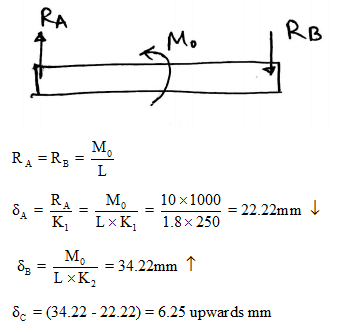## GATE Civil Engineering (CE) 2023 Mock Test Series

27 docs|296 tests
Information about Practice Test: Civil Engineering (CE)- 4 Page
In this test you can find the Exam questions for Practice Test: Civil Engineering (CE)- 4 solved & explained in the simplest way possible. Besides giving Questions and answers for Practice Test: Civil Engineering (CE)- 4, EduRev gives you an ample number of Online tests for practice

## GATE Civil Engineering (CE) 2023 Mock Test Series

27 docs|296 tests(Scan QR code)